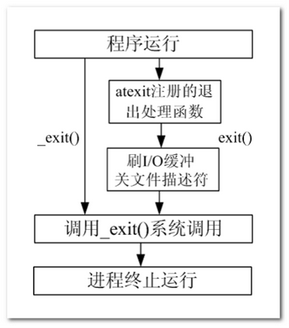# exit

• #include <stdlib.h>

• value：返回给父进程的参数（低 8 位有效），至于这个参数是多少根据需要来填写。

# _exit()

• #include <unistd.h>

exit()_exit() 函数功能和用法是一样的，无非时所包含的头文件不一样，还有的区别就是：exit()属于标准库函数，_exit()属于系统调用函数。#include<stdio.h>
#include <stdlib.h>
#include <unistd.h>

int main(){
printf("Hello world");//没有换行符，不会刷新缓冲区
exit(0);  //会输出上述文字
//_exit(0); //不会输出上述文字
while(1);  //不让子进程结束
return 0;
}


return的话就不做过多解释了，C语言里面应该很好理解。

# wait函数

• 阻塞等待子进程退出
• 回收子进程残留资源
• 获取子进程退出状态（退出原因）

• #include <sys/types.h>
• #include <sys/wait.h>

• status：传出参数，回收进程的状态。

WIFEXITED(status) --> 为真 -->调用 WEXITSTATUS(status) --> 得到 子进程 退出值。

WIFSIGNALED(status) --> 为真 -->调用 WTERMSIG(status) --> 得到 导致子进程异常终止的信号编号。

• 成功：返回回收的子进程pid
• 失败：返回 -1， 设置errno

# waitpid

• pid：指定回收某一个子进程pid，可选项如下：
• -1：代表任意回收一个子进程
• 0：待回收的子进程的pid

• 0：同组的子进程
• status：指定回收某一个子进程pid
• options：指定回收的方式
• WNOHANG： 非阻塞回收
• NULL：阻塞回收

• 0 : 表成功回收的子进程 pid

• =0 : 函数调用时， 参3 指定了WNOHANG， 并且，没有子进程结束。
• -1: 失败。errno

# 案例

int main(){
pid_t pid;
pid = fork();

if(pid < 0){
perror("fork");
exit(0);
} else if(pid == 0) {
int i = 0;
for(i = 0;i < 5;i++){
printf("I am child pid is %d\n",getpid());
sleep(1);
}
exit(2);
} else {
int status;
pid_t pid1 = wait(&status); //阻塞回收
if(WIFEXITED(status)){
printf("son process return %d pid is %d\n", WEXITSTATUS(status), pid1);
}
printf("This is parent\n");
}
return 0;
}#include<stdio.h>
#include <stdlib.h>
#include <unistd.h>
#include <sys/wait.h>

int main(){
pid_t pid, tmppid;
int i = 0;
for(i = 0;i < 5;i++){
pid = fork();
if(pid == 0)
break;
if(i == 2)
tmppid = pid;
}

if(i == 5){
sleep(5);
int status;
pid_t pid1;
//waitpid(-1, &status, 0); // 和 wait() 没区别，0：阻塞
//waitpid(pid, &status, 0); // 指定等待进程号为 pid 的子进程， 0 阻塞
pid1 = waitpid(tmppid, &status, WNOHANG);//// WNOHANG：不阻塞

printf("This is parent waitpid is %d\n", pid1);

} else {
sleep(i);
printf("I am %dth child pid is %d\n", i+1, getpid());
}
return 0;
}## 补充

wait、waitpid 一次调用，回收一个子进程，想要回收多个必须使用 while 循环

#include<stdio.h>
#include <stdlib.h>
#include <unistd.h>
#include <sys/wait.h>

int main(){
pid_t pid;
int i = 0;
for(i = 0;i < 5;i++){
pid = fork();
if(pid == 0)
break;
}

if(i == 5){
pid_t pid1;
while( pid1 = wait(NULL)){
if(pid1 == -1)
break;
printf("This is parent waitpid is %d\n", pid1);
}
} else {
printf("I am %dth child pid is %d\n", i+1, getpid());
}
return 0;
}